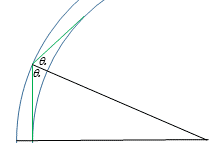# Internal Reflection / Fiber Optics

• jfulky

## Homework Statement

3. A fiber optic cable is essentially a thin fiber of plastic with n = 1.46, surrounded by a protective material with n = 1.32. The light can travel around a bend in the fiber if it can internally reflect inside the fiber. If the diameter of the fiber is d = 0.900 mm find the smallest inner radius of curvature, R, into which the fiber can be bent that will allow light that is initially traveling parallel to the fiber to remain inside the fiber.

Sin(Θ)c = n1/n2

## The Attempt at a Solution

My work is in the thumbnail. I am not entirely sure how to approach this problem. My though process was to find the critical angle in which the light is internally reflected, and then use geometry to find the radius of curvature, since I am not sure of any more equations that would help me. I also though of using arc length in some way, to relate the distance the light traveled in the tube to the circumference of the circle.

Any help would be appreciated!

## Homework Statement

3. A fiber optic cable is essentially a thin fiber of plastic with n = 1.46, surrounded by a protective material with n = 1.32. The light can travel around a bend in the fiber if it can internally reflect inside the fiber. If the diameter of the fiber is d = 0.900 mm find the smallest inner radius of curvature, R, into which the fiber can be bent that will allow light that is initially traveling parallel to the fiber to remain inside the fiber.

Sin(Θ)c = n1/n2

## The Attempt at a Solution

My work is in the thumbnail. I am not entirely sure how to approach this problem. My though process was to find the critical angle in which the light is internally reflected, and then use geometry to find the radius of curvature, since I am not sure of any more equations that would help me. I also though of using arc length in some way, to relate the distance the light traveled in the tube to the circumference of the circle.

Any help would be appreciated!
• You analyzed a light ray starting in the middle of the fiber optic cable. I do not recommend using a a light ray starting in the middle of the cable. Instead, let the light ray start out tangent to the inner radius of the bent cable -- not in the middle of the cable, but rather just touching (and tangent to) the inner side of the cable.
• In your diagram, you have the entire 90 deg bend accomplished by a single reflection; in other words you drew $\theta_C = 45^\circ$. This is not only unrealistic, but it will add confusion later down the road. In a realistic cable, there will be many reflections down the road to achieve a total $90^\circ$ bend. Also, as part of the process of solving this, there will be (depending on your approach) a different right triangle involved, even if it's only a small part of the overall bend. I don't want to confuse this right triangle with any other possible right triangles.

Start with drawing a small section of the cable such that there are parts of two concentric circles. The light ray starts out vertical, as you have already drawn it, except it starts out tangent to the inner radius. Then let the light ray follow a straight line until it reflects off of the outer radius, and then comes back to being tangent once again with the inner radius.

But don't force $\theta_C = 45^\circ$. It's okay to allow it to be a larger angle than that.

Now you can use geometry to solve for one of the radii. (And if you solved it the way I did, there will be a right triangle that fits in there somewhere.)Edit: Just to be clear, start with a figure like something along these lines:Last edited:
Thank you for the explanation, the problem is actually extremely simple, I don't know why I missed that insight! As a side note: when dealing with light ray problems, is it reasonable to align the light ray in any way you want that simplifies the problem? I sometimes struggle with what to assume the light ray's path is.

•collinsmark
Thank you for the explanation, the problem is actually extremely simple, I don't know why I missed that insight! As a side note: when dealing with light ray problems, is it reasonable to align the light ray in any way you want that simplifies the problem? I sometimes struggle with what to assume the light ray's path is.
I suggested aligning the light ray tangent to the inner radius because it is the extremum (i.e., it is an extreme case).

Whenever the problem asks you to look for "maximum <something>" or "minimum <something>", it's an indication that you might want to consider aligning the light ray in one of its exreme cases.[Edit: that is: an extremum that makes logical sense; you don't have to worry about aligning the light ray into physically impossible configurations, but you do want to look for cases at the physical limits.]